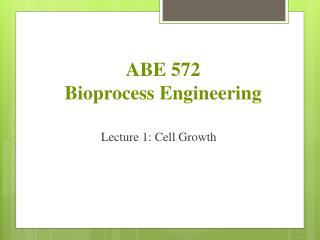DownloadDownload PresentationABE 572 Bioprocess Engineering

# ABE 572 Bioprocess Engineering

Télécharger la présentation## ABE 572 Bioprocess Engineering

- - - - - - - - - - - - - - - - - - - - - - - - - - - E N D - - - - - - - - - - - - - - - - - - - - - - - - - - -
##### Presentation Transcript

1. ABE 572Bioprocess Engineering Lecture 1: Cell Growth

2. Phases of cell growth Cell growth can be modelled with four different phases: • Lag phase: Cells adapt themselves to growth conditions. It is the period where the individual bacteriaare maturing and not yet able to divide. • Exponential phase(log phase) is a period characterized by cell doubling.The number of new bacteria appearing per unit time is proportional to the present population.

3. Stationary phase is often due to a growth-limiting factor such as the depletion of an essential nutrient, and/or the formation of an inhibitory product such as an organic acid. Stationary phase results from a situation in which growth rate and death rate are equal. • At death phase, bacteria run out of nutrients and die.

4. Cell growth in bioreactor

5. If μ is constant w.r.t., then rearranging previous equation and integrating, we get: or or This equation applies only to the duration of the exponential growth phase, beyond which either substrate limitation or toxin accumulation become rate determining.

6. Doubling Time • It is the time required to double the quantity of biomass, that is growing exponentially. • X: X0 2X0 (The amount of biomass at the start must double) t: 0  td (Within a finite time, td, or doubling time) Substituting in 2X0 for X and td for t and logging both sides => , therefore • Specific growth rate (μ) can be defined as any point during the growth cycle. During the exponential growth period is constant and at a maximum for that process under the specified conditions.

7. Next lecture • Chemostat • Fed-Batch Reactor